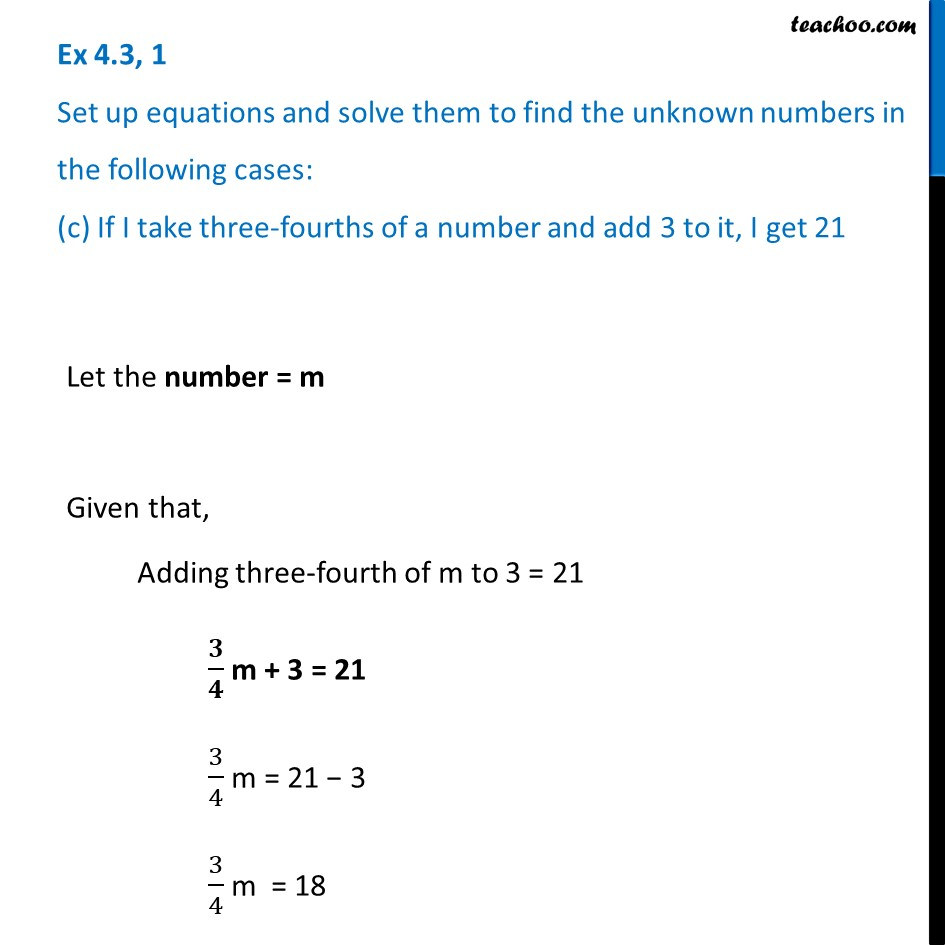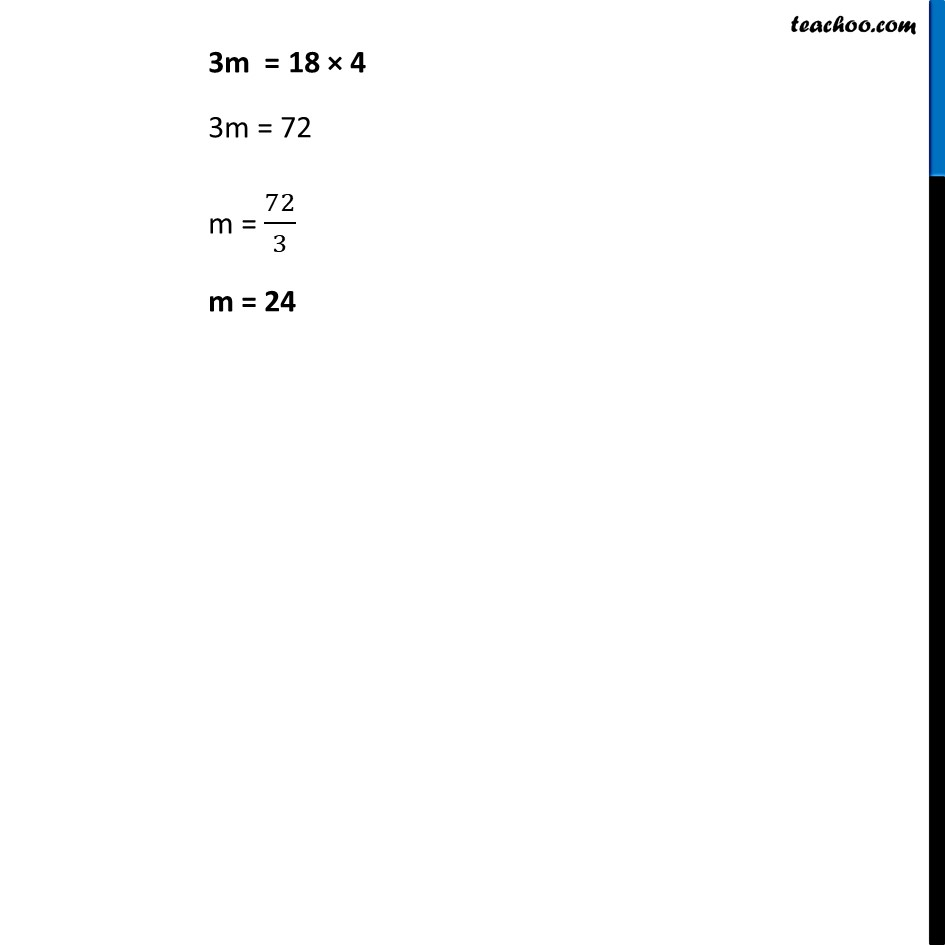Ex 4.3

Chapter 4 Class 7 Simple Equations
Serial order wiseLearn in your speed, with individual attention - Teachoo Maths 1-on-1 Class

### Transcript

Ex 4.3, 1 Set up equations and solve them to find the unknown numbers in the following cases: (c) If I take three-fourths of a number and add 3 to it, I get 21Let the number = m Given that, Adding three-fourth of m to 3 = 21 𝟑/𝟒 m + 3 = 21 3/4 m = 21 − 3 3/4 m = 18 3m = 18 × 4 3m = 72 m = 72/3 m = 24